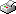Print This Page Feng Li -- doctoral thesis -- Bayesian Modeling of Conditional Densities Abstract This thesis develops models and associated Bayesian inference methods for flexible univariate and multivariate conditional density estimation. The models are flexible in the sense that they can capture widely differing shapes of the data. The estimation methods are specifically designed to achieve flexibility while still avoiding overfitting. The models are flexible both for a given covariate value, but also across covariate space. A key contribution of this thesis is that it provides general approaches of density estimation with highly efficient Markov chain Monte Carlo methods. The methods are illustrated on several challenging non-linear and non-normal datasets. In the first paper, a general model is proposed for flexibly estimating the density of a continuous response variable conditional on a possibly high-dimensional set of covariates. The model is a finite mixture of asymmetric student-t densities with covariate-dependent mixture weights. The four parameters of the components, the mean, degrees of freedom, scale and skewness, are all modeled as functions of the covariates. The second paper explores how well a smooth mixture of symmetric components can capture skewed data. Simulations and applications on real data show that including covariate-dependent skewness in the components can lead to substantially improved performance on skewed data, often using a much smaller number of components. We also introduce smooth mixtures of gamma and log-normal components to model positively-valued response variables. In the third paper we propose a multivariate Gaussian surface regression model that combines both additive splines and interactive splines, and a highly efficient MCMC algorithm that updates all the multi-dimensional knot locations jointly. We use shrinkage priors to avoid overfitting with different estimated shrinkage factors for the additive and surface part of the model, and also different shrinkage parameters for the different response variables. In the last paper we present a general Bayesian approach for directly modeling dependencies between variables as function of explanatory variables in a flexible copula context. In particular, the Joe-Clayton copula is extended to have covariate-dependent tail dependence and correlations. Posterior inference is carried out using a novel and efficient simulation method. The appendix of the thesis documents the computational implementation details. Key words: Bayesian inference; density estimation; smooth mixtures; surface regression; copulas; Markov chain Monte Carlo. ISBN 978-91-7447-665-1